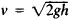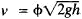# Torricelli's Law

Also found in: Wikipedia.
The following article is from The Great Soviet Encyclopedia (1979). It might be outdated or ideologically biased.

## Torricelli’s Law

(or Torricelli’s theorem), a statement of the speed of efflux of a liquid from an orifice in an open container. In equation form the law is, where h is the height of the free surface of the liquid above the center of the orifice and g is the acceleration of gravity. The law was discovered by E. Torricelli in 1641.

It follows from Torricelli’s law that the speed of efflux of a liquid from an orifice is the same for all liquids and depends solely on the height from which the liquid falls. In other words, the speed is equal to that attained by a body falling freely from the height h.

The actual speed of efflux differs somewhat from that given by Torricelli’s law and depends on the shape and size of the orifice; the viscosity of the liquid; and the flow rate, or discharge. To take these factors into account, a correction coefficient is introduced, and the equation given above then takes on the form. The value of φ is less than unity. For small circular orifices and high Reynolds numbers φ is equal to 0.94–0.99. Values of φ for orifices of other shapes and sizes are given in hydraulics handbooks.